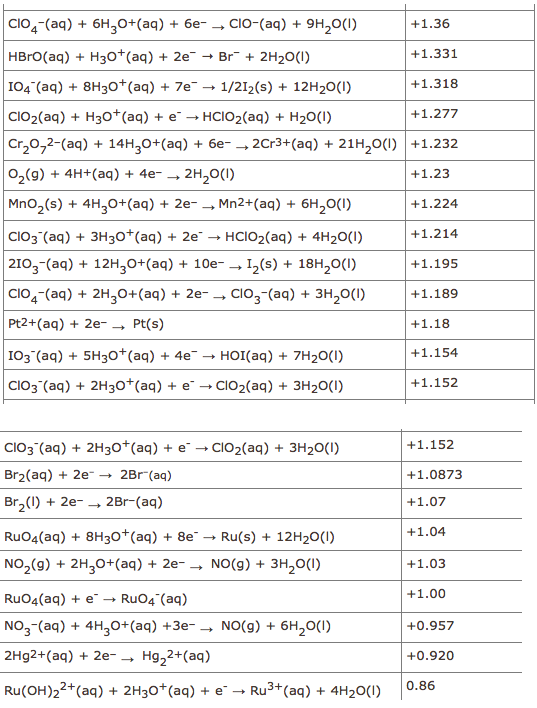# Problem: Calculate the E°cell for the following equation. Use these standard potentials .ClO4- (aq) + 6H3O+ (aq) + 6Br- (aq)  →  3Br2 (aq) + ClO- (aq) + 9H2O (l)

###### FREE Expert Solution
83% (131 ratings)
###### Problem Details

Calculate the E°cell for the following equation. Use these standard potentials .

ClO4(aq) + 6H3O(aq) + 6Br(aq)  →  3Br(aq) + ClO(aq) + 9H2O (l)What scientific concept do you need to know in order to solve this problem?

Our tutors have indicated that to solve this problem you will need to apply the Cell Potential concept. If you need more Cell Potential practice, you can also practice Cell Potential practice problems.

What is the difficulty of this problem?

Our tutors rated the difficulty ofCalculate the E°cell for the following equation. Use these s...as high difficulty.

How long does this problem take to solve?

Our expert Chemistry tutor, Dasha took 3 minutes and 36 seconds to solve this problem. You can follow their steps in the video explanation above.

What professor is this problem relevant for?

Based on our data, we think this problem is relevant for Professor Varghese's class at GENESEO.